1.* (1997 F 11I) The elementary steps of a reaction (with their rate constants) are as follows:

A + B --k1--> C
C --k2--> D
D --k3-->B + E

What is the reaction? What is the rate of appearance of product E?

2.* (1997 3 5) The gas reaction N2O5 --> 2NO2 + 1/2O2 follows the Arrhenius equation k = Aexp(-Ea/RT) where R = 8.314J K-1 mol-1 and 1 calorie = 4.184J. At the temperature T=273K, k=0.0887 x 10-5 s-1, whereas at T = 298K, k = 3.46 x 10-5 s-1. What are the values of the activation energy Ea in kcal/mol and the pre-exponential factor A is s-1?

3.* (1996 F 16) At an altitude of 30 km and a latitude of 00 the atmospheric temperature is 233 K. The concentration of ozone and oxygen atoms are chlorine atoms are 2 x 1012 molecules/cm3, 1 x 108 atoms/cm3, and 1 x 105 atoms/cm3, respectively. Under these conditions the following reactions occur, each of which obey the Arrhenius rate equation: k=Aexp(-Ea/RT):

 Reaction Log10A Ea/R O3 + Cl --> O2 + ClO -10.82 2218 ClO + O3 --> O2 + O2 + Cl -10.55 257 ClO + O --> O2+ Cl -10.11 130

Where the units are cm3molecule-1s-1for A and K for Ea/R.

A. Write down a differential equation for the disappearance of ozone from the first reaction.

B. Find the rate for ozone dissapearance from reaction 1.

C. Write down a differential equation for the disappearance of ozone from the second reaction.

D. Find the rate for ozone disappearance from the second reaction.

E. Use the information you have to estimate the steady-state concentration of ClO.

F. What is the role of Cl in the second and third reactions

G. How does the rate of destruction of ozone from the first reaction compare to the rate of destruction from the second and third reactions?

4.* (1995 F 9) A worker in a dry cleaning plant is exposed through inhalation to halogenated hydrocarbons that bind strongly to the same liver enzyme (alcohol dehydrogenase) that catalyzes the oxidation of ethanol (C2H5OH) to acetaldehyde (CH3CHO). These halohydrocarbons persist in the liver for several days. Suppose one evening this worker walks out of the plant with 80% of the liver enzyme sites occupied by halohydrocarbons and is invited by a friend to "have a few beers" before dinner. Please recall the homework problem on hyman alcoholysis in which you learned that the zero-order rate constant for oxidation of ethanol in a fully functioning liver is 0.17 mole/hr and that one bottle of ber contains 0.34 moles of ethanol. Provide this worker with an estimate of how many bottles of beer he can drink and be fully sober (free of ethanol) by the start of the next morning (15 hours later).

5.* (1995 F 11) In many contamination processes, the contaminating species A dissappears by first-order kinetics with a rate constant k in the process given by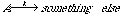.

A. Suppose that a constant quantity DA of the contaminant A is added at regular intervals of time Dt starting at time t=0. Show that the contaminant in the system builds up in time as the sum of the geometrical progression
[A(t)]=
DA (1+ x + x2 + x3 + x4 . . .) (1)
where
x=exp(-k
Dt) (2)

B. The following information is useful. The sum of the series is
[An]=
DA(1-xn+1)/(1-x) (3)
after a time t=n
Dt (4)

As n --> infinity, Eq. 3 becomes
[A(infinite time)]=
DA/(1-x) (5)

Suppose that a freshman, dismayed by the dorm food offerings, elects to eat 200 g of tuna fish every day. Suppose that the tuna fish provided by Food Service contains 1.0 part per million by weight of mercury. The half-life for elimination of mercury from the body is 70 days, and the toxic threshold where symptoms of mercury poisoning appear is 0.014g. Calculate the grams of mercury in our tuna addict at the end of freshman year, taken as 240 days. Is our tuna-fish-salad lover in any danger of becoming sick?

6.* (1995 3 5B) Is the approach of the concetration of a reagent A to equilibrium always monotonically decreasing if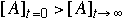?

7.* (1995 3 6) The reaction between bromate ions and bromide ions in acidic aqueous solution is

BrO3-(aq) + 5Br- (aq) + 6H+ (aq) --> 3Br2(l) + 3H2O(l)

Using the data from 4 experiments shown below determine the orders of the rate of reaction for all three reactants, the overall reaction order, and the value of the rate constant k.

Experiment[BrO3-] (M) [Br-] (M)[H+] (M)rate (M/s)
1
0.100.100.108.0 x 10-4
2
0.200.100.101.6 x 10-3
3
0.200.200.103.2 x 10-3
4
0.100.100.203.2 x 10-3

8.* (1994 F 4) Metal surfaces often serve as catalysts. For example on a hot copper surface, ethanol (C2H5OH) decomposes to acetaldehyde (CH3CHO) and hydrogen (H2). Experimentally, it is found that at low concentrations of ethanol vapor the decomposition rate is proportional to the ethanol vapor concentration whereas at high concentrations the rate becomes independent of the ethanol vapor concentration.

A. Write a three-step mechanism for the ethanol decomposition process. Use for your notation: A = ethanol; S = available catalytic sites on the copper surface; AS = ethanol - surface site complex; B = products.

B. Derive from this mechanism the rate law for the decomposition of ethanol on a hot copper surface and show how it can account for the observations mentioned above concerning the dependence of the decomposition rate on ethanol vapor concentration. In addition to the steady-state approximation, you will want to introduce a constraint that arises because the total number S0 of catalytic sites is fixed.

9.* (1994 F 5) The thermal decomposition of acetaldehyde (CH3CHO) was measured at two temperatures. At T=700K, the rate of this second-order process was 0.011 (mol/L)-1s-1and at T=760K it was 0.105 (mol/L) -1s-1.

Use this data to estimate the activation energy Ea and the pre-exponential factor A given that the universal gas constant R has the value 8.314 JK-1mol-1. State clearly the units of Ea and A.

10.* (1994 3 7) Consider two consecutive first-order reactions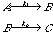in which the reactant A is transformed into the product C via the intermediate B. Many examples of this behavior are found in radioactive decay processes in which the intermediate is also radioactive (unstable). The rate equation for the disappearance of the reactant A is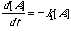(1a)

The rate equation for the appearance of the product C is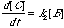(1b)

A. Write down an expression for the time rate of change of the concentration of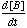in terms of the two first-order rate constants k1 and k2.

B. Differentiate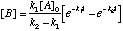with respect to time and compare it with your result from part A.

C. How does the [B] vary with time? Under what conditions does [B] show a maximum value?

11.* (1993 3 4) The development of a photographic mage on film is a process controlled by kinetics. Exposed silver halide crystals in the film are reduced to silver in a developing solution. The development process is performed for a certain amount of time, which depends on the temperature of the developing solution, and with agitation to ensure transfer of reagents to the film.

A. The time required for development at a particular temperature is inversely proportional to the rate constant for the process (assume k = 1/t).

The following table gives the development times (in minutes) for Eastman Kodak’s Tri-x film in two types of developing solution.

 Developer 180C 200C 210C 220C 240C T-max 7 min 6 6 5.5 5.5 D-76 10 min 9 8 7 6

Use the Arrhenius equation to determine the activation energy (in Kcal/mol) for the development process using D-76 developer. (R = 1.986 cal mol-1 K-1)

B. Film development is a first order process, and for Tri-x is 45% complete after 4 min. at 20šC using D-76 developing solution. What is the rate constant for this process, and what is the half life?

C. Increasing the temperature speeds the rate at which the film is developed. There are, of course, limits to the temperatures which can be used. For example, at elevated temperatures the emulsion on the film begins to degrade, resulting in a "grainy" picture. The maximum temperature of development is defined as the temperature at which the rate of degradation exceeds the rate of development. For Tri-x film the activation energy for the emulsion degradation process is 19.86 Kcal/mol with a preexponential factor of 3 x 1013 s-1.

Which developer (T-max or D-76) will allow us to develop Tri-x film the most rapidly? Explain.

D. What is the time? (Hint: use your graph from part A)